# Calculation of Motor Power Dissipation for Different Motion Profiles

Symbols:

X = Distance to travel in meter
T1 = Acceleration time in second
T2 = Coasting time in second
T3 = Deceleration time in second
T4 = Stop time in second
T = T1 + T2 + T3 = Time to move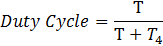VPeak = Peak velocity in meter / second
a1 = Acceleration in meter / second2
a3 = Deceleration in meter / second2
F1 = Force during acceleration in Newton
F2 = Force during coasting
F3 = Force during deceleration in Newton
F4 = Force during stop time
Frms = The Root Mean Square (RMS) of the force
m = Total moving mass, mass of the motor + all other masses attached to it in Kg
Irms = The Root Mean Square (RMS) of current in Amp
Kf = Force constant in Newton / Amp
Ke = BEMF constant in Volts / meter / second
R = Resistance of the motor in Ohm
f = Frequency in Hz
P = Power dissipation in Watt

#### 1.  Trapezoidal Velocity Profile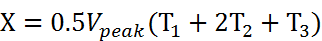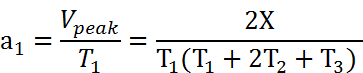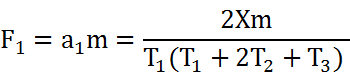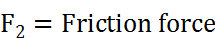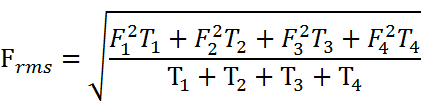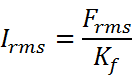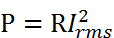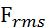Should be equal or less than the CONTINUOUS FORCE of the motor.

P should be equal or less than the MAX CONTINOUS POWER of the motor.

####